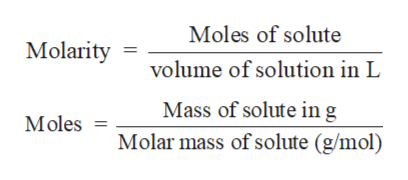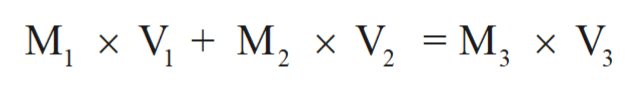# What is the final concentration of HCl in a solution prepared by addition of 922.0 mL of 4.73 M HCl to 549.0 mL of 2.03 M HCl?  Assume volumes are additive.

Question
139 views

What is the final concentration of HCl in a solution prepared by addition of 922.0 mL of 4.73 M HCl to 549.0 mL of 2.03 M HCl?  Assume volumes are additive.

check_circle

Step 1

Solution stoichiometry involves the calculation of concentration of solutions in the given conditions of volumes, moles etc.

Solution stoichiometry is mainly based on the calculation of moles and volumes. These two values are used to calculate the molarity of solution. The relation between moles, volume and molarity is as given below;help_outlineImage TranscriptioncloseMoles of solute Molarity volume of solution in L Mass of solute ing Molar mass of solute (g/mol) Moles fullscreen
Step 2

The molarity of mixture of two solutions can be calculated with the help of:help_outlineImage Transcriptioncloseм, xV, + M, х V, - м, х V, V2 M X 3 fullscreen
Step 3

Given:

V1 =  922.0 mL

M1 = 4.73 M

V2 = 549.0 mL

M2 = 2.03 M

M3 = Need to calculate

V3= 92...

### Want to see the full answer?

See Solution

#### Want to see this answer and more?

Solutions are written by subject experts who are available 24/7. Questions are typically answered within 1 hour.*

See Solution
*Response times may vary by subject and question.
Tagged in

### Solutions